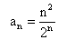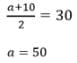# Test: Introduction To Sequences

## 10 Questions MCQ Test Mathematics For JEE | Test: Introduction To Sequences

Description
Attempt Test: Introduction To Sequences | 10 questions in 10 minutes | Mock test for JEE preparation | Free important questions MCQ to study Mathematics For JEE for JEE Exam | Download free PDF with solutions
QUESTION: 1

### The 5th term of the sequenceis

Solution:

an = (n2)/2n
⇒ a5 = [(5)2]/2(5)
⇒ a5 = 25/32

QUESTION: 2

### A sequence is a function whose domain is the set of

Solution:

The correct option is A.

A sequence is an enumerated collection of objects in which repetitions are allowed and order does matter. Like a set, it contains members. The number of elements is called the length of the sequence. A sequence is a function whose domain is the set of natural numbers or a subset of the natural numbers.

QUESTION: 3

### The arithmetic mean between a and 10 is 30, the value of ‘a’ should be

Solution:QUESTION: 4

The first 4 terms of the sequence a1 = 2, an = 2an-1 + 1 for n > 2 are

Solution:

a1 = 2
a2 = 2a1 + 1
=> 2(2) + 1 = 5
a3 = 2a2 + 1
=> 2(5) + 1 = 11
a4 = 2a3 + 1
=> 2(11) + 1 = 23
Hence, the required series is : 2,5,11,23………

QUESTION: 5

What is the 10th term of the sequence defined by an = (n-1)(2-n)(3+n)?

Solution:

an = (n-1)(2-n)(3+n)
Put n = 10
an = 9×(-8)×13
= - 936

QUESTION: 6

The 10th term of the sequence an = 2(n -1)(2n - 1) is

Solution:

an = 2(n -1)(2n - 1)
a10 = 2(10-1)(2(10)-1))
= 2(9)(19)
= 342

QUESTION: 7

The sum of the series for the sequence an = (2n-1)/2, for 1 < n < 5 is

Solution:

Put n=1 then a1=1/2

then put n=2 a2=3/2

put n=3 a3=5/2

n=4 a4=7/2

n=5 a5=9/2

their sum is 25/2

QUESTION: 8

7th term of Geometric Progression 2, 6, 18, ... is

Solution:

How do we get from 2 to 6? One way is to multiply by 3.

How do we get from 6 to 18? We can multiply by 3 once again.

What about 18 to 54? Again, we can multiply by 3.

We notice that our common ratio is 3. We can leverage this fact to write the next terms of our sequence:

...54,(54⋅3),(54⋅32),(54⋅33)

Notice, we are multiplying by three every time. The 7th term of this sequence is given by the blue expression

54⋅33, which is equal to

54⋅27=1458

QUESTION: 9

The sequence whose terms follow the certain pattern is called a

Solution:

Those sequences whose terms follow certain patterns are called progressions

QUESTION: 10

A sequence in which (any term) − (its immediate previous term) gives a constant is called

Solution:

An arithmetic sequence is a list of numbers with a definite pattern. If you take any number in the sequence then subtract it by the previous one, and the result is always the same or constant.Use Code STAYHOME200 and get INR 200 additional OFF Use Coupon Code